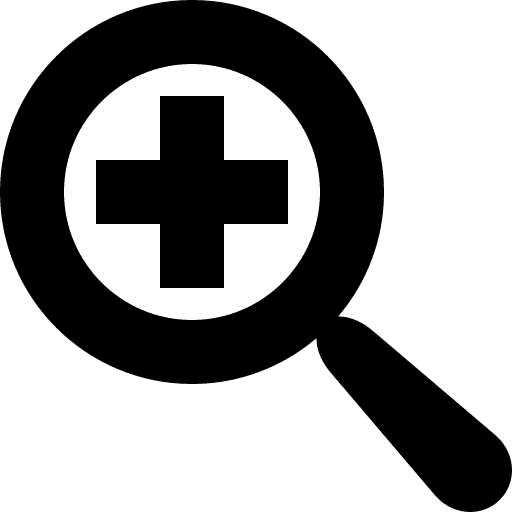My trial lesson

How Much Money Do I Have?

What are our 4 main coins?
What do they look like?
How much are they worth?
1 / 25
Slide 1: Slide

This lesson contains 25 slides, with interactive quizzes and text slides.

Items in this lesson

How Much Money Do I Have?

What are our 4 main coins?
What do they look like?
How much are they worth?

U.S. Coins!

Slide 2 -Mind map

Quarter = 25 cents

Dime = 10 cents

Nickel = 5 cents

Penny = 1 cent

Slide 7 -Slide

Which coin is missing?

Slide 8 -Open question

25 + 25 + 10 + 10 + 1 = 71 cents

Slide 9 -Slide

25 + 25 + 10 + 10 + 10 + 10 + 10

5 + 1 = 6 cents

Slide 11 -Slide

What does a quarter look like?

Slide 12 -Open question

If you have 2 quarters, how many cents do you have?
A
20 cents
B
15 cents
C
2 cents
D
50 cents

Slide 13 -Quiz

What does a dime look like?

Slide 14 -Open question

Which coin is the biggest?
A
Dime
B
Nickel
C
Quarter
D
Penny

Slide 15 -Quiz

I have 75 cents, so I must have....
A
3 quarters
B
2 quarters, 2 dimes and 1 nickel
C
2 quarters, 1 dime, 2 nickels, and 5 pennies
D
1 quarter and 5 dimes

Slide 16 -Quiz

What does a penny look like?

Slide 17 -Open question

If you have 2 pennies and 3 nickels, how much money do you have?
A
18 cents
B
17 cents
C
12 cents
D
62 cents

Slide 18 -Quiz

What does a nickel look like?

Slide 19 -Open question

How much money do I have?
A
12 cents
B
41 cents
C
45 cents
D
32 cents

Slide 20 -Quiz

I have 32 cents, so I have...
A
2 quarters and 1 penny
B
4 dimes and 2 nickels
C
3 dimes and 2 pennies
D
1 quarter, 1 nickel and 2 pennies

Slide 21 -Quiz

2 quarters, 3 dimes, 1 nickel and 2 pennies..... how much money is this?

Slide 22 -Open question

I have 12 cents... they look like this!
ABCDSlide 23 -Quiz

Each coin is different!

Slide 24 -Slide

Money Matters!

Why do we have coins?

Who counts coins?

Where would we count coins?